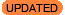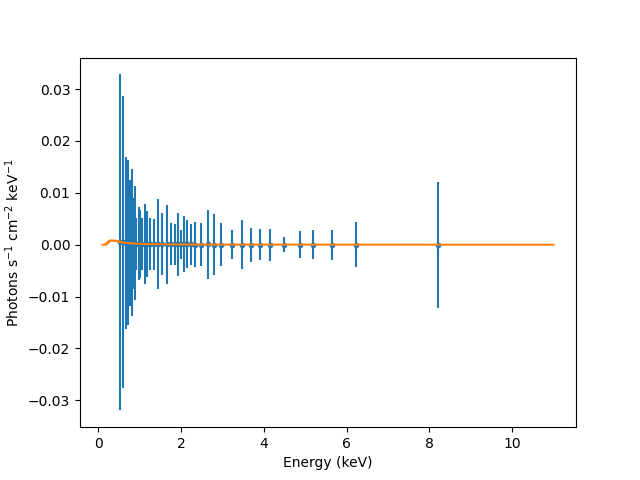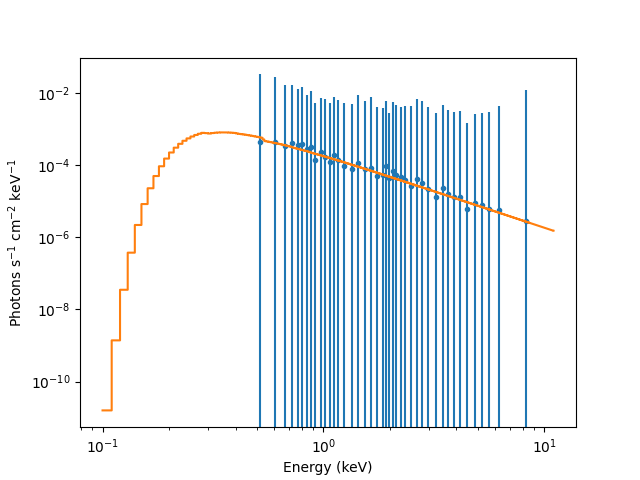# How can I plot a model-fitted spectrum in photon flux units (photons s-1 cm-2 keV-1)?Warning

Earlier versions of this entry had two issues:

• evaluation of the source expression
• no normalization by the bin width

which could cause problems depending on exactly what model components were used in the model expression (although in some cases they would roughly cancel each other out).

In Sherpa 4.14 - when using PHA data - model-fitted source spectra are plotted in units of counts s-1keV-1 (or per channel or wavelength). This is because Sherpa convolves an assumed source model in photon flux units with the instrument response functions to get a model-predicted flux in counts s-1keV-1, so that this model may be compared to a data set in counts. To plot in photon flux units (photons s-1 cm-2 keV-1) would require doing things the other way around - it requires deconvolving the data using the RMF and ARF instrument responses and comparing it to a model which is unconvolved (i.e., the model representation of source flux before it passes through the detector).

The following set of Sherpa 4.14 commands may be used to plot a model-fitted source spectrum in photon flux units. (To unfold a source spectrum independent of a model, see Sherpa Plotting FAQ #3.)Note

Please note that the following produces only an approximation of the deconvolved source data.

It also requires that the "analysis settings" set by set_analysis are at their default values of:

• quantity="energy"
• type="rate"
• factor=0

which can be checked with the following (change 1 to the correct dataset identifier if necessary and the rate setting of True is equivalent to setting type="rate" in the set_analysis call):

```sherpa> get_analysis(1)
'energy'
sherpa> get_data(1).rate
True
sherpa> get_data(1).plot_fac
0
```

First we start by accessing the data used to create the plot_fit plot, which contains information on the "data" and "model" components:

```sherpa> fplot = get_fit_plot()
sherpa> dplot = fplot.dataplot
sherpa> mplot = fplot.modelplot
```

We can evaluate the source model on the grid used for the fit (this gives the predicted emission before it enters the telescope and interacts with the mirrors and detector):

```sherpa> mdl = get_source()
sherpa> src = mdl(dplot.xlo, dplot.xhi)  # use the low and high edges here, not the mid-point
```

The aim is to plot the "fluxed" data in units of photon / cm2 / s, but the current values and their types are:

Array Units
dplot.x, dplot.xlo, dplot.xhi keV
dplot.y, mplot.y count / s / keV
src photon / cm2 / s

The predicted per-bin values (mplot.y) can be used to "correct" the observed data points (dplot.y) using the evaluated source expression (src):

```sherpa> conv = src / mplot.y / (dplot.xhi - dplot.xlo)  # divide by the bin width
```

which creates a value with the units photon / count / cm2, which can be applied the existing data (and it's error) to create a new dataset, labelled "fluxed":

```sherpa> yconv = conv * dplot.y
sherpa> dyconv = conv * dplot.yerr
sherpa> load_arrays("fluxed", dplot.x, yconv, dyconv, Data1D)
```

With this, we can now plot the "fluxed" data set and overplot the source model on it:

```sherpa> plot_data('fluxed')
sherpa> plot_source(overplot=True)
sherpa> plt.xlabel('Energy (keV)')
sherpa> plt.ylabel('Photons s\$^{-1}\$ cm\$^{-2}\$ keV\$^{-1}\$')
```

The plotted values in Figure 1 represent the ratio of the source count rate to the convolved model count rate, times the unconvolved model (photons s-1 cm-2 kev-1).

### Linear axes### Linear axes

and then with logarithmically-scaled axes in Figure 2:

```sherpa> plt.xscale('log')
sherpa> plt.yscale('log')
```

### Log axes### Background

The primary function of software packages like Sherpa is to solve the integral equation (instrument response) which relates the total counts produced in a given pulse-height bin in a spectrum to the incident source spectrum. Therefore, once you have a pulse-height spectrum and the appropriate RMF and ARF instrument response, you can use Sherpa to fold the source data through an assumed model to determine the "true" source flux (see also the Sherpa function calc_photon_flux, which returns non-integrated flux in units of photons s-1 cm-2 keV-1 when supplied with a single energy value, and when working in energy space with set_analysis("energy")).

Though be warned that Sherpa has no explicit knowledge of data or model units; the units displayed with computed fluxes are defaults, generally correct for standard analyses of 1-D PHA energy/wavelength spectra (XSPEC-like analyses). They may be incorrect for non-standard analyses, or for analyses of 2-D spatial images with exposure maps, etc. The correct units can be determined by working backwards from the data, taking into account the exposure time, the units of the instrument model, the bin units, etc.